# Unit of Resistance

## Introduction

Resistance plays a major role in the study of electricity. Electricity is so important for various applications in our daily life. Electric current, which is the flow of electrons, passes through the electric circuit with the component’s resistor, capacitor, inductors are used. The electrical component resistor is used in the circuit to allow current of a particular amount and stops the remaining.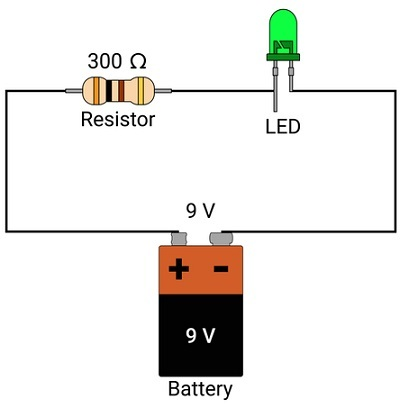This was done by the circuit element called resistor by producing some resistance in the circuit.

## What is Resistance?

We know a conductor material produces electric current by movement of free electrons in a particular direction when voltage is applied to the conductor. In order to control the flow of current, some resistance is produced within the material. Hence, resistance is the variable parameter which opposes the flow of current when a voltage is applied across an electric circuit. It is denoted by R. Resistor is the device that produces resistance in an electric circuit.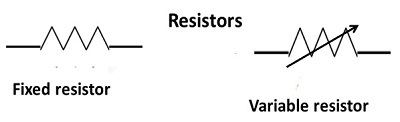• Fixed resistor: It is a linear resistor with a fixed resistance value.

• Variable Resistor: It is a resistor which contains various resistance values. This can be obtained by varying the current in the electric circuit with voltage constant. The variable resistor is also called Rheostat.

## Factors affecting Resistance:

There are four factors that affect the resistance of a resistor.

• Depends on the nature of the material the resistor is made up of. For example, the material is a conductor made up of copper, gold etc. Then it contains less resistance due to high conduction of free electrons and hence produces more electric current. If the material is an insulator made up of rubber, plastic etc exhibits less conduction and hence their resistance will be low. Semiconductors produce very high resistance than conductors and insulators.

• Depends on Temperature: If the temperature of the material or the resistor increases, there will be an increase in resistance. If $\mathrm{R_0}$ is the resistance at $\mathrm{0^0 C}$ temperature and $\mathrm{R_f}$ is the final resistance at $\mathrm{t^0 C}$ temperature then equation for the final resistance $\mathrm{R_f}$ is,

$$\mathrm{R_f =R_0(1+\alpha t+\beta t^2) }$$

$\mathrm{\alpha}$ and $\mathrm{\beta}$ are the temperature coefficient of resistances that vary for different materials and their unit is $\mathrm{\frac{1}{k}}$ or $\mathrm{\frac{1}{t^0 C}}$

• Depends on the length of the material used in the resistor. If the length of the material increases, the resistance also increases. It means they both are directly proportional to each other. i.e.,

$$\mathrm{R\:\alpha\:l}$$

Here l is the length of the material.

For example, if there is a long wire of conductor material connected to the electric circuit, then due to more space there will be an increase in flow of electrons and also collisions between them that opposes the current flow and vice versa.

• Depends on the area of the cross section of the material. If the area of the material increases, the resistance decreases. It means they both are inversely proportional to each other. i.e.,

$$\mathrm{R\:\alpha\frac{1}{A}}$$

A is the area of the cross section of the material. Thicker the material less will be the resistance and thinner the material high will be the resistance.

For example, if the area of cross section of a conductor material connected to the electric circuit is high, it means wider space, then the flow of electrons will be at high rate which can overcome the resistance in the circuit. Hence, the resistance will decrease.

## Unit of Resistance

The S.I unit of resistance is ‘ohm’, which is named after a German physicist and mathematics scientist Georg Simon Ohm. Its symbol is $\mathrm{\Omega}$.

## Ohm’s Law

Important variable parameters in electrical circuits or devices are current, voltage and resistance. Georg Simon Ohm while studying electric current passing through conductors, he found that the current variable increases due to increase in voltage. It means that both variables are directly proportional to each other when the temperature variable is constant. i.e.,

$$\mathrm{V\:\alpha\:I}$$

For removing the proportionality, we need a constant,

$$\mathrm{V\:=IR}$$

This equation is called Ohm’s law and here, R is that proportionality constant, which is called Resistance.

Hence, the resistance R, by using ohm’s law is −

$$\mathrm{R =\frac{V}{I}}$$

By this the unit of resistance ohm can also be written as, $\mathrm{ohm=\frac{Volt}{ampere}}$ i.e.,

If a 1 volt voltage is applied to an electric circuit for a material then 1 ampere current is produced in it with 1 ohm resistance.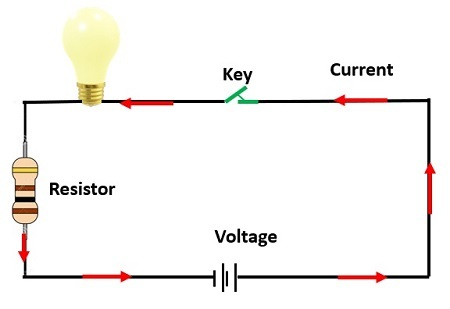## What is Resistivity?

We know from the topic, factors affecting resistance, length and area of the conductor are directly and inversely proportional to the resistance. i.e,

$$\mathrm{R\:\alpha\:l\:\:and\:\:R\:\alpha\:\frac{1}{A}}$$

Combining both the resistance R mathematically can be written as,

$$\mathrm{R\:\alpha\:\frac{l}{A}}$$

For removing the proportionality, we need a constant,

$$\mathrm{R\:=\rho\:\frac{l}{A}}$$

Here, ρ is that proportionality constant, which is called Resistivity or specific resistance of the conductor.

For finding S.I units of resistivity, rewrite the above equation for resistivity.

$$\mathrm{\rho=\frac{RA}{l}\:\Rightarrow\:\rho=\frac{1\:\Omega\:1 m^2}{1m}\:\Rightarrow\:\rho=\Omega\:m}$$

For a conductor of 1 m length and $\mathrm{1m^2}$ area then the resistance produced by the conductor will be 1Ω m. Resistivity measures the resistance limit of any material produced for a given length and area cross section of that material.

## Conclusion

In this tutorial we learned about resistance and its units. Resistance is the property of materials that opposes the electric current in the electric circuit and its unit is ohm (Ω) . Ohm’s law gives relation between voltage, current and resistance. Resistivity is the parameter which gives the amount of resistance offered by the conductors. Good conductors exhibit less resistivity value and increase the flow of electrons leading to good conduction of electricity.

## FAQs

Q1. What are the devices that are helpful in measuring resistance and voltage?

Ans. The device used to measure resistance and voltage is voltmeter or multimeter. Ammeter is used for measuring current produced in an electric circuit.

Q2. If two or three resistors are connected series in an electric circuit, what will be their relation?

Ans. When the resistors are connected in series like shown in the figure, then the combined resistance value is,

$$\mathrm{R_{total} = R_1+R_2+R_3}$$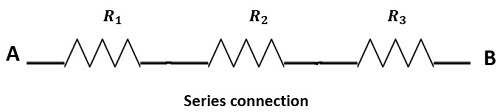Q3. If two or three resistors are connected parallel in an electric circuit, what will be their relation?

Ans. When the resistors are connected in parallel like shown in the figure, then the combined resistance value is,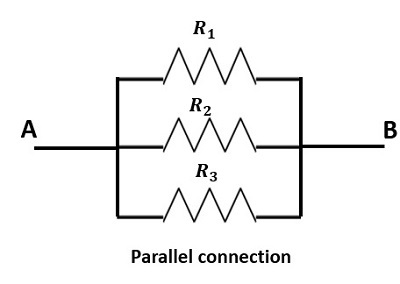Q4. Give resistivity values for some metals at a constant temperature?

Ans. The resistivity values of copper, aluminium, tungsten, and iron at $\mathrm{20^{\circ} C}$ temperature is,

• Copper =$\mathrm{1.72\times 10^{−8}\:\Omega\:m}$

• Aluminium =$\mathrm{ 2.65\times 10^{−8}\:\Omega\:m}$

• Tungsten =$\mathrm{5.65\times 10^{−8}\:\Omega\:m}$

• Iron = $\mathrm{9.71\times 10^{−8}\:\Omega\:m}$

Q5. Write any two or three sources of errors while measuring the resistance in an electric circuit?

Ans. The three major errors occur while measuring the resistance are

• If there is any leakage of the electric current in the circuit.

• While note down the measuring values in the multimeter like round off values.

• Due to surrounding disturbances around the electric circuit, like wind, humidity etc.

Updated on: 07-Apr-2023

270 Views